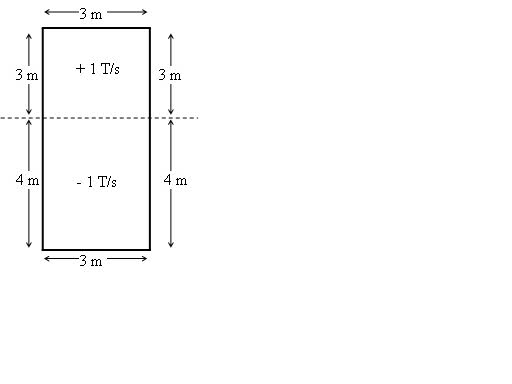# Net force on loop in B field

## Homework Statement

The figure shows a 3.00 meter by 7.00 meter rectangular loop of wire with resistivity 3.90x10-6 Ohm-m and a resistance of 25.0 Ohms. Initially, the magnetic field is zero everywhere. However, after t = 0, B increases at 1.00 T/s out of the page everywhere above the dotted line, and increases at 1.00 T/s into the page everywhere below the dotted line.What is the net force on the loop at t = 2.00 seconds? Be sure to indicate the net direction

F = IlB
I = E/R = BA/Rt

## The Attempt at a Solution

I figured out the current in the loop and got I = .12A CCW

I'm not sure what to do about the force though. First off, the induced E is the same at every second, right? Second, what length do you use? I know if you had a bar or something moving you would use the length of the bar that lies in the B field, but no bar is moving here. And last but not least, how can you tell the direction of the force? I'm pretty sure the current would be equal at every part of the loop, so is the net force just 0? Thanks for the help :D

Last edited:

The net change in flux with respect to time is a constant, so the induced emf is constant as well. That is how you got a constant current.

You wrote down the correct force equation, and the direction is solved using the right-hand-rule. You have 4 sides of the rectangle, so check the net force at each side. Be careful with the left and right sides of the rectangle since there will be two forces you calculate. And direction does matter.

Note also that the magnetic field is changing. So find the magnetic field after 2 seconds first. Then do all the force calculations and directions.

The net change in flux with respect to time is a constant, so the induced emf is constant as well. That is how you got a constant current.

You wrote down the correct force equation, and the direction is solved using the right-hand-rule. You have 4 sides of the rectangle, so check the net force at each side. Be careful with the left and right sides of the rectangle since there will be two forces you calculate. And direction does matter.

Note also that the magnetic field is changing. So find the magnetic field after 2 seconds first. Then do all the force calculations and directions.
Since the current is going to be the same everywhere in the loop, don't all the forces just cancel out?

That is true if the magnetic field was the same everywhere. Even though the magnitude of the magnetic field is changing, you still know its direction so you can apply the right-hand-rule and find the direction of the force vectors.

For example, check the direction of the forces for the top and bottom wires of the loop.

Wow, for some reason I'm really confused. The force they're talking about is from the magnetic field, right? You do right hand rule I induced crossed into magnetic field, right?

The force is from the magnetic field. If you have a current carrying wire in a magnetic field. You can use your right hand to find the force the magnetic field exerts on the wire.

Opening your hand out completely flat. Your fingers are in the direction of the magnetic field, and the thumb is in the direction of the current. Then the force is in the direction of the palm of your hand.

Ok, so for the left and right sides of the top square, 3m part: those forces cancel because they are both pointing out.

For the left/right sides of the 4m part: F = (.12)(4)(2)= .96N (pointing in for both sides, so they add?)

For the top/bottom: F = (.12)(3)(2) = .72N (up for both of them)

So net F is 2(.96) towards center and 2(.72N) up?

Well the bottom left/right sides both point in opposite directions so they cancel as well when you add them.

You are right about the forces from the top and bottom wires since they both point in the same direction.

Oh ok. I don't know why, but I had a major brain fart concerning forces pointing in. For some reason I thought they added. Thanks for all the help!

Just one more quick semi-related question: so if you have a square loop in a magnetic field pointing into the page and increasing, the net force on the loop is still 0?

Right, since all the force vectors would point in opposite directions and have the same magnitudes.

Ok cool, appreciate it :D